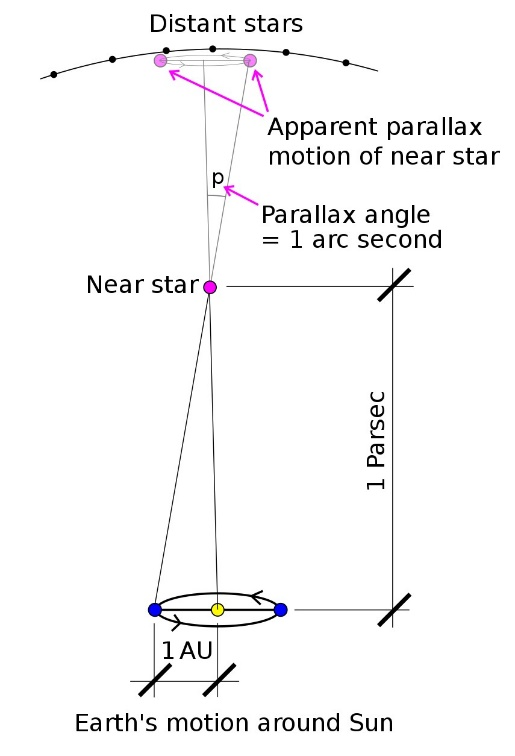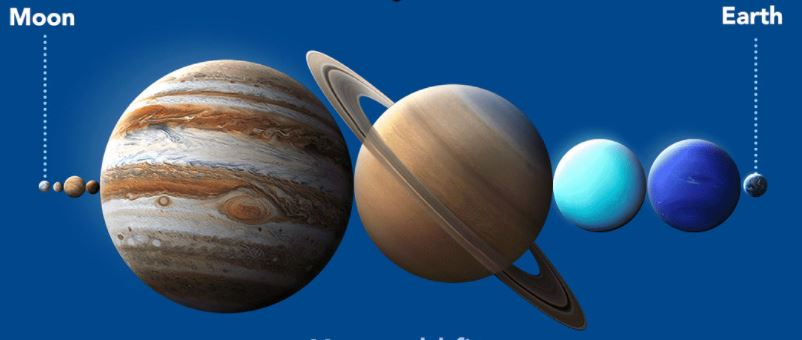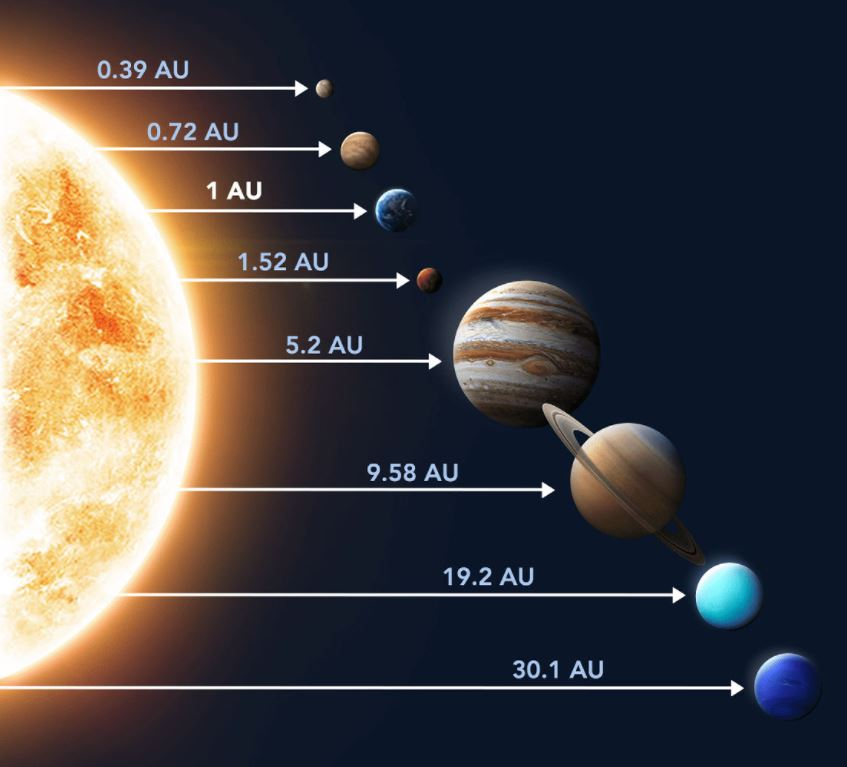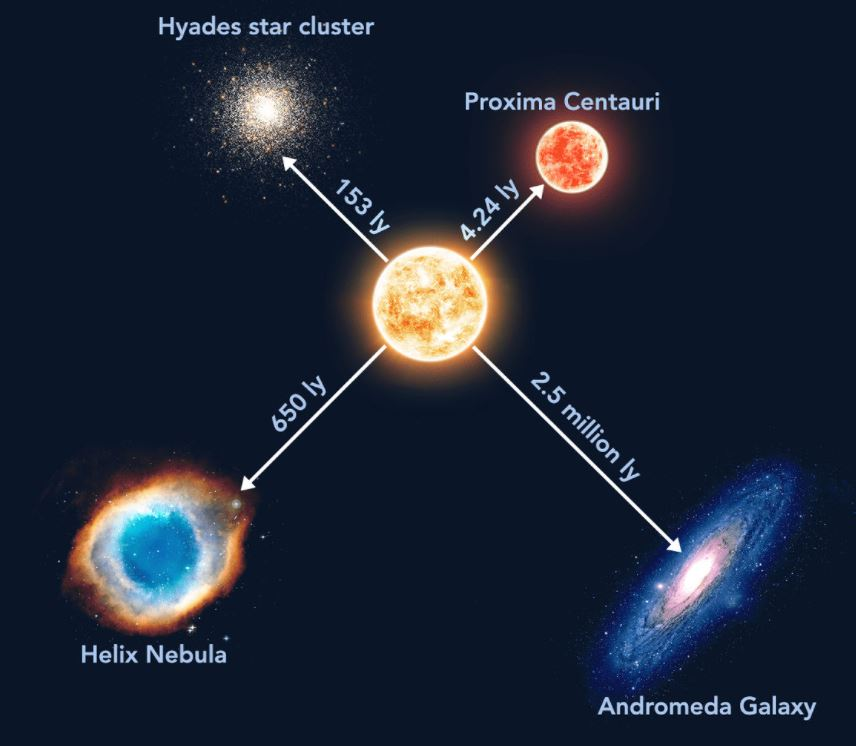USE PROMO CODE "DEMO100" TO AVAIL FREE DEMO CLASSMeasuring distances in space

Space is very vast, even our solar system is so vast that to explore it with our current technology would take decades and a lot of resources. If we use the normal units to measure the distance between planets and the star, we will have to write thousands and millions of kilometres each time we need it. So, the astronomers defined new sets of units to measure distance of the heavenly bodies. They are all mentioned below.

Lunar Distance (LD)

The instantaneous Earth–Moon distance, or distance to the Moon, is the distance from the centre of Earth to the centre of the Moon. The LD is a unit of measure in astronomy. More technically, it is the semi major axis of the geocentric lunar orbit.The lunar distance is approximately 384,400 km. this is roughly 30 times earth’s diameter. The actual distance varies over the course of the orbit of the Moon, from 356,500 km at the perigee to 406,700 km at apogee, resulting in a differential range of 50,200 km.

Lunar distance is commonly used to express the distance to near-Earth object encounters. Lunar semi-major axis is an important astronomical datum; the few millimetres precision of the range measurements determines semi-major axis to a few decimetres; it has implications for testing gravitational theories such as general relativity, and for refining other astronomical values such as Earth mass, Earth radius, and Earth's rotation. The measurement is also useful in characterizing the lunar radius, the mass of the Sun and the distance to the Sun.

Astronomical unit (AU)

It is roughly the distance between earth and the Sun and equal to about 150 million kilometres. The actual distance varies by about 3 percent as earth orbits the sun from a maximum (aphelion) to a minimum (perihelion) and back again once year. The astronomical unit was originally conceived as the average of Earth's aphelion and perihelion; however, since 2012 it has been defined as exactly 149597870700 m.The astronomical unit is used primarily for measuring distances within the Solar System or around other stars. It is also a fundamental component in the definition of another unit of astronomical length, the parsec.

The parsec (symbol: pc) is a unit of length used to measure the large distances to astronomical objects outside the Solar System, approximately equal to 3.26 light-years or 206,000 astronomical units (au), i.e., 30.9 trillion kilometres. Parsec is obtained using parallax and trigonometry and is defined as the distance at which 1 au subtends an angle of one arcsecond.The nearest star, Proxima Centauri, is about 1.3 parsecs (4.2 light-years) from the Sun. Most of the stars visible to the unaided eye in the night sky are within 500 parsecs of the Sun. The word parsec is a portmanteau of "parallax of one second" and was coined by the British astronomer Herbert Hall Turner in 1913 to make calculations of astronomical distances from only raw observational data easy for astronomers. Partly for this reason, it is the unit preferred in astronomy and astrophysics, though the light-year remains prominent in popular science texts and common usage.One could fit all the other planets in the solar system between earth and the moonThe distance of various planets from the Sun

Light Year (LY)

The light-year, alternatively spelled lightyear, is a unit of length used to express astronomical distances and is equivalent to about 9.46 trillion kilometres (9.46×1012 km). As defined by the International Astronomical Union (IAU), a light-year is the distance that light travels in vacuum in one Julian year (365.25 days).Because it includes the word "year", the term light-year is sometimes misinterpreted as a unit of time.

The light-year is most often used when expressing distances to stars and other distances on a galactic scale, especially in non-specialist contexts and popular science publications. The unit most used in professional astronomy is the parsec (symbol: pc, about 3.26 light-years) which derives from astrometry.Distances expressed in light-years include those between stars in the same general area, such as those belonging to the same spiral arm or globular cluster. Galaxies themselves span from a few thousand to a few hundred thousand light-years in diameter and are separated from neighbouring galaxies and galaxy clusters by millions of light-years. Distances to objects such as quasars and the Sloan Great Wall run up into the billions of light-years.The distance between star clusters and galaxy